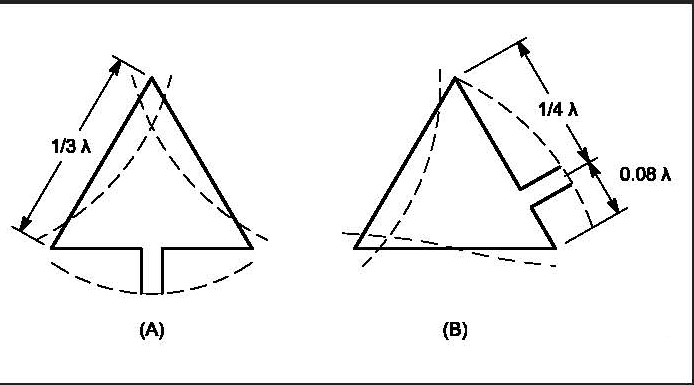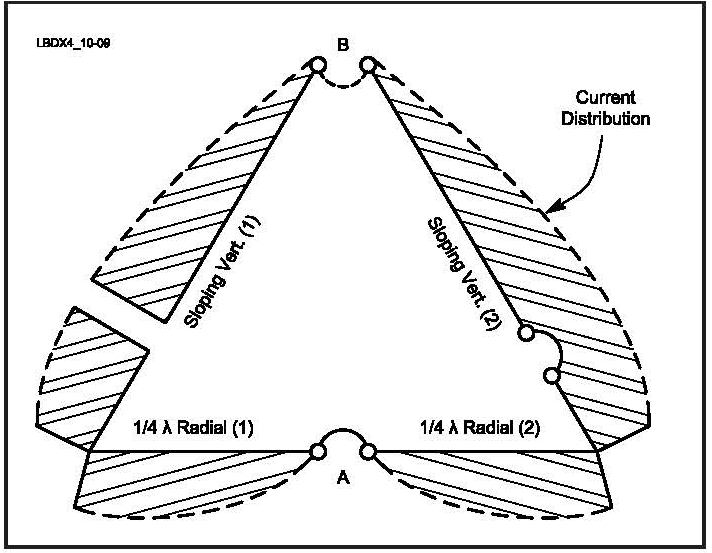# VERTICAL DELTA LOOPS

#### Just as the inverted-V dipole has been described as the poor man’s dipole, the delta loop can be called the poor man’s quad loop. Because of its shape, the delta loop with the apex on top is a very popular antenna for the low bands; it needs only one support. In free space the equilateral triangle produces the highest gain and the highest radiation resistance for a three-sided loop configuration. As we deviate from an equilateral triangle toward a triangle with a long baseline, the effective gain and the radiation resistance of the loop will decrease for a bottom­ corner-fed delta loop. In the extreme case (where the height of the triangle is reduced to zero), the loop has become a half­ wavelength-long transmission line that is shorted at the end, which shows a zero-Ω input impedance (radiation resistance), and thus zero radiation (well-balanced open-wire line does not radiate). Just as with the quad loop, we can switch from horizontal to vertical polarization by changing the position of the feed point on the loop. For horizontal polarization the loop is fed either at the center of the baseline or at the top of the loop. For vertical polarization the loop should be fed on one of the sloping sides, at λ/4 from the apex of the delta. Fig 10-8 shows the current distribution in both cases.Fig 10-8—Current distribution for equilateral delta loops fed for (A) horizontal and (B) vertical polarization.

# 2.1. Vertical Polarization

### 2.1.1. How it works

#### Assume we construct the antenna with the center of the horizontal bottom wire open. Now we can see the two half baselines as two λ/4 radials, one of which provides the necessary low-impedance point for connecting the shield of the coax. The other radial is connected to the bottom of the second sloping vertical, which is the other sloping wire of the delta loop. This is similar to the situation encountered with a λ/4 vertical using a single elevated radial (see Chapter 9 on vertical antennas). The current distribution in the two quar­ter-wave radials is such that all radiation from these radials is effectively canceled. The same situation exists with the voltage-fed T antenna, where we use a half-wave flat top (equals two λ/4 radials) to provide the necessary low-imped­ ance point to raise the current maximum to the top of the T antenna.The vertically polarized delta loop is really an array of two λ/4 verticals, with the high-current points spaced 0.25 λFig 10-9—The delta loop can be seen as two λ/4 sloping verticals, each using one radial. Because of the current distribution in the radials, the radiation from the radials is effectively canceled.

For more on this see ON4UN Low-Band DXing

Credits ON4UN Low - Band DXing

Loop Antennas Full Chapter Here